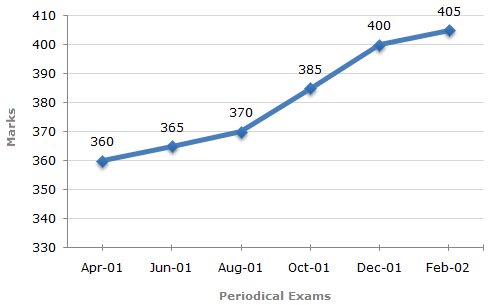# Data Interpretation - Line Charts - Discussion

### Discussion :: Line Charts - Line Chart 10 (Q.No.3)

In a school the periodical examination are held every second month. In a session during April 2001 - March 2002, a student of Class IX appeared for each of the periodical exams. The aggregate marks obtained by him in each perodical exam are represented in the line-graph given below.

Marks Obtained by student in Six Periodical Held in Every Two Months During the Year in the Session 2001 - 2002.

Maximum Total Marks in each Periodical Exam = 5003.

What is the percentage of marks obtained by the student in the periodical exams of August, 01 and Oct, 01 taken together ?

 [A]. 73.25% [B]. 75.5% [C]. 77% [D]. 78.75%

Explanation:

 Required percentage =(370 + 385) x 100% =755 x 100% = 75.5%. (500 + 500) 1000

 Obi said: (Mar 20, 2013) Where is the 1000 coming from please I need answer?

 Sowmiya said: (Jun 9, 2013) 1000 Comes, Because Maximum Total Marks in each Periodical Exam = 500.

 Sayak Halder said: (Aug 3, 2019) How come 500+500?

 Nidhirvyay said: (May 10, 2021) How come 500 + 500? Explain me.